# 你没听过的梅森旋转算法

（标准开头）

（算法本身挺学术的，我努力写得轻松点）

# 旋转算法简介

###### 可能有的同学对这个循环节有点质疑。可能觉得2^19937-1有点短？

219937数量级是106001（梅森镇楼）

->continue

# 前置知识

（用词不准确轻喷）

#### 线性反馈移位寄存器（LFSR）（看到这里应该还没懵吧）

->continue

# 原理分析1. 初始数组 { 1 ， 0 ， 0 ， 0 } （为什么不是 0，0，0，0 你们可以自己想想，文章末尾揭晓）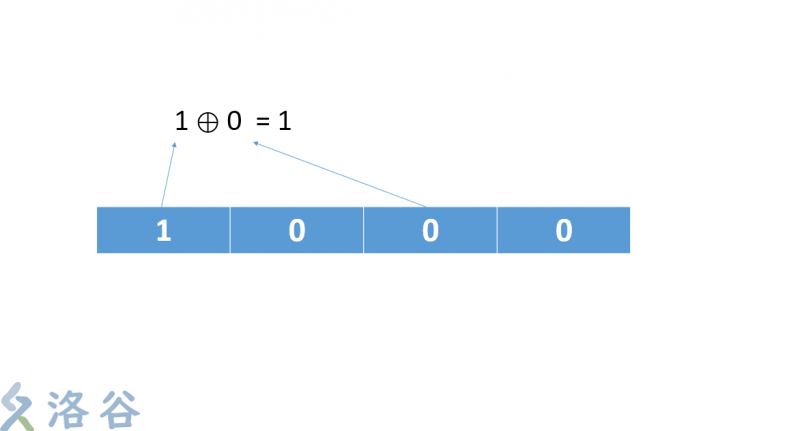1. 将它的第四位和第二位抓出来做异或运算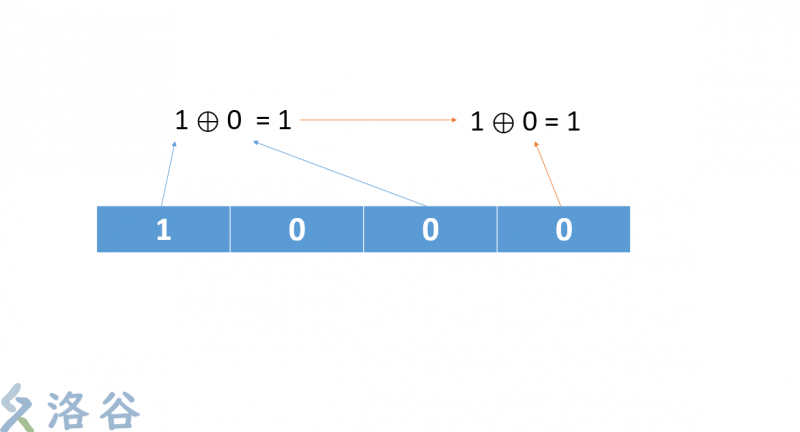1. 把刚刚的运算结果和最后一位再做一次运算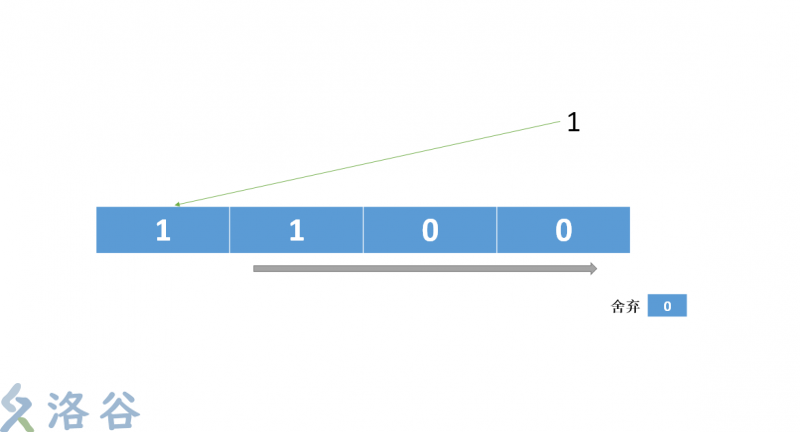1. 把最后的运算结果放到第一位，序列后移。最后一位被无情的抛弃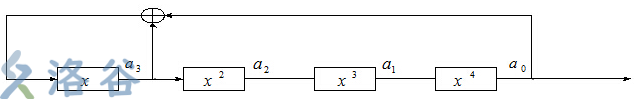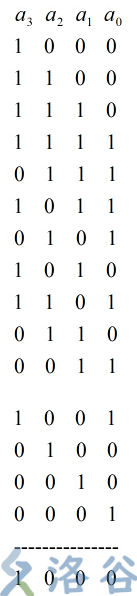（图片摘自原文链接

#### 关于旋转

（本来想做gif的，后来不知道怎么做出旋转）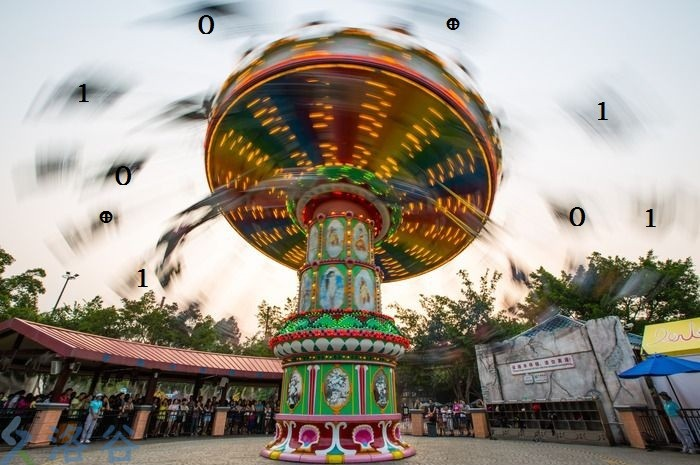->continue

# 代码实现

（笔者很懒，直接搬原代码出处的代码）

#include <iostream>
#include <string.h>
#include <stdio.h>
#include <time.h>

using namespace std;

bool isInit;
int index;
int MT;  //624 * 32 - 31 = 19937

void srand(int seed)
{
index = 0;
isInit = 1;
MT = seed;
for(int i=1; i<624; i++)
{
int t = 1812433253 * (MT[i-1] ^ (MT[i-1] >> 30)) + i;
MT[i] = t & 0xffffffff;   //取最后的32位
}
}

void generate()
{
for(int i=0; i<624; i++)
{
// 2^31 = 0x80000000
// 2^31-1 = 0x7fffffff
int y = (MT[i] & 0x80000000) + (MT[(i+1) % 624] & 0x7fffffff);
MT[i] = MT[(i + 397) % 624] ^ (y >> 1);
if (y & 1)
MT[i] ^= 2567483615;
}
}
int rand()
{
if(!isInit)
srand((int)time(NULL));
if(index == 0)
generate();
int y = MT[index];
y = y ^ (y >> 11);
y = y ^ ((y << 7) & 2636928640);
y = y ^ ((y << 15) & 4022730752);
y = y ^ (y >> 18);
index = (index + 1) % 624;
return y;  //笔者注：y即为产生的随机数
}

int main()
{
srand(0);  //设置随机种子
int cnt = 0;
for(int i=0; i<1000000; i++)  //下面的循环是用来判断随机数的奇偶概率的
{
if(rand() & 1)
cnt++;
}
cout<<cnt / 10000.0<<"%"<<endl;
return 0;
}


->continue

# 填一下前面的坑

（* o *）

（ ⊕ o ⊕ )

posted @ 2020-01-24 18:45  CHNmuxii  阅读(...)  评论(...编辑  收藏